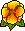## Singularities of eddy current problems

Martin Costabel, Monique Dauge, Serge Nicaise 

Articles and SlidesWe consider the time-harmonic eddy current problem in its electric formulation. By proving the convergence in energy, we justify in what sense this problem is the limit of a family of Maxwell transmission problems: Rather than a low frequency limit, this limit has to be understood in the sense of Bossavit, that is, when the ratio

conductivity / (permittivity x frequency) ----> infinity.

We describe the singularities of the solutions when the conductor is a polyhedral domain. They are related to edge and corner singularities of certain problems for the scalar Laplace operator, namely the interior Neumann problem, the exterior Dirichlet problem, and possibly, an interface problem. These singularities are the limit of the singularities of the related family of Maxwell problems.In a SECOND PAPER, we concentrate on the uniform behavior of conical singularities with respect to the small parameter characterizing the eddy current limit.Preprint at the Newton Institute Cambridge: NI03019
Link to the Preprint Webpage of the Newton InstitutePreprint, pdf (220 k)

Published in Math. Model. Anal. Num. (M2AN) 37, 5 807-831.Slides on Maxwell and eddy current singularities, and convergence to the eddy current model (Oberwolfach, Feb. 2004).Slides, pdf (116 k)